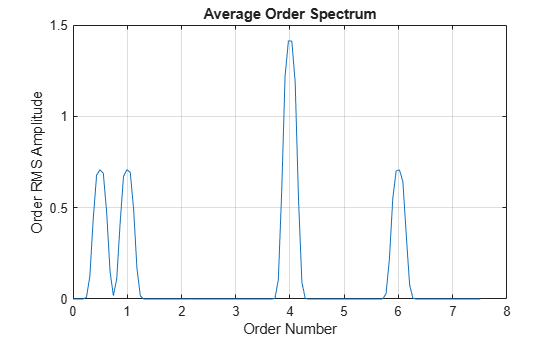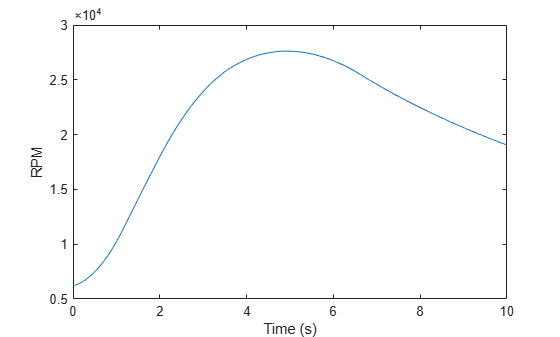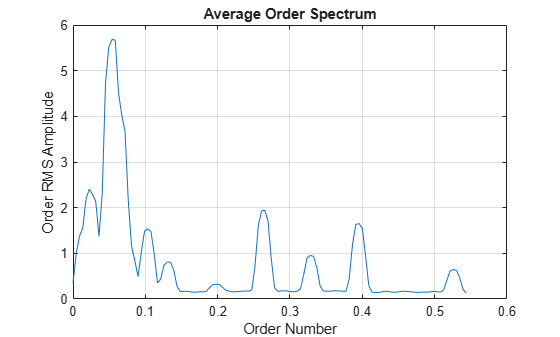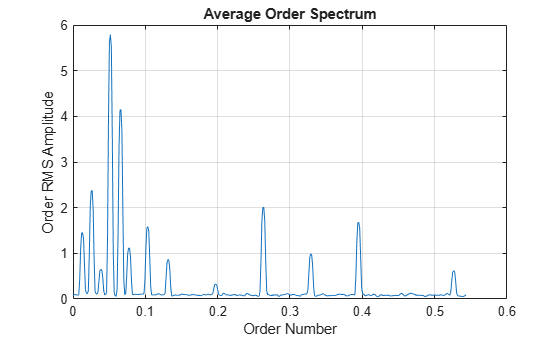orderspectrum

Average spectrum versus order for vibration signal

Description

example

spec = orderspectrum(x,fs,rpm) computes an average order-magnitude spectrum vector, spec, for an input signal, x, sampled at a rate of fs Hz. To compute the spectrum, orderspectrum windows a constant-phase, resampled version of x with a flat top window.

[spec,order] = orderspectrum(x,fs,rpm) also returns a vector of the orders corresponding to each average spectrum value.

example

[spec,order] = orderspectrum(map,order) computes an average order-magnitude spectrum vector starting from an order-RPM map and a vector of orders. Use rpmordermap to compute map and order. map must be linearly scaled. The returned amplitudes are the same as in map. The returned spectrum is scaled linearly.

[spec,order] = orderspectrum(map,order,'Amplitude',amp) specifies the type of amplitude to consider when computing an average order-magnitude spectrum starting from an order-RPM map.

orderspectrum(___) with no output arguments plots the RMS amplitude of the order spectrum, scaled linearly, on the current figure.

Examples

collapse all

Create a simulated signal sampled at 600 Hz for 5 seconds. The system that is being tested increases its rotational speed from 10 to 40 revolutions per second during the observation period.

fs = 600;
t1 = 5;
t = 0:1/fs:t1;

f0 = 10;
f1 = 40;
rpm = 60*linspace(f0,f1,length(t));

The signal consists of four harmonically related chirps with orders 1, 0.5, 4, and 6. The order-4 chirp has twice the amplitude of the others. To generate the chirps, use the trapezoidal rule to express the phase as the integral of the rotational speed.

o1 = 1;
o2 = 0.5;
o3 = 4;
o4 = 6;

ph = 2*pi*cumtrapz(rpm/60)/fs;

x = [1 1 2 1]*cos([o1 o2 o3 o4]'*ph);

Visualize the order-RPM map of the signal.

rpmordermap(x,fs,rpm)Visualize the average order spectrum of the signal. The peaks of the spectrum correspond to the ridges seen in the order-RPM map.

orderspectrum(x,fs,rpm)Analyze simulated data from an accelerometer placed in the cockpit of a helicopter.

Load the helicopter data. The vibrational measurements, vib, are sampled at a rate of 500 Hz for 10 seconds. The data has a linear trend. Remove the trend to prevent it from degrading the quality of the order estimation.

vib = detrend(vib);

Plot the nonlinear RPM profile. The rotor runs up until it reaches a maximum rotational speed of about 27,600 revolutions per minute and then coasts down.

plot(t,rpm)
xlabel('Time (s)')
ylabel('RPM')Compute the average order spectrum of the signal. Use the default order resolution.

orderspectrum(vib,fs,rpm)Use rpmordermap to repeat the computation with a finer order resolution. The lower orders are resolved more clearly.

[map,order] = rpmordermap(vib,fs,rpm,0.005);

orderspectrum(map,order)Compute the power level for each estimated order. Display the result in decibels.

[map,order] = rpmordermap(vib,fs,rpm,0.005,'Amplitude','power');

spec = orderspectrum(map,order);

plot(order,pow2db(spec))
xlabel('Order Number')
ylabel('Order Power Amplitude (dB)')
grid onInput Arguments

collapse all

Input signal, specified as a row or column vector.

Example: cos(pi/4*(0:159))+randn(1,160) specifies a sinusoid embedded in white Gaussian noise.

Sample rate, specified as a positive scalar expressed in Hz.

Rotational speeds, specified as a vector of positive values expressed in revolutions per minute. rpm must have the same length as x.

• If you have a tachometer pulse signal, use tachorpm to extract rpm directly.

• If you do not have a tachometer pulse signal, use rpmtrack to extract rpm from a vibration signal.

Example: 100:10:3000 specifies that a system rotates initially at 100 revolutions per minute and runs up to 3000 revolutions per minute in increments of 10.

Order-RPM map, specified as a matrix. Use rpmordermap to compute order-RPM maps.

Orders in order-RPM map syntax, specified as a vector. The length of order must equal the number of rows in map.

Order-RPM map amplitudes, specified as one of 'rms', 'peak', or 'power'.

• 'rms' — Assumes that the order-RPM map uses the root-mean-square amplitude for each estimated order.

• 'peak' — Assumes that the order-RPM map uses the peak amplitude for each estimated order.

• 'power' — Assumes that the order-RPM map uses the power level for each estimated order.

Output Arguments

collapse all

Average order-magnitude spectrum, returned as a vector of root-mean-square (RMS) amplitudes in linear scale. If you use map and order as input arguments, and set 'Amplitude' to 'power' when using rpmordermap to compute map, then orderspectrum returns spec in power units.

Output orders, returned as a real vector.

 Brandt, Anders. Noise and Vibration Analysis: Signal Analysis and Experimental Procedures. Chichester, UK: John Wiley & Sons, 2011.

 Vold, Håvard, and Jan Leuridan. “High Resolution Order Tracking at Extreme Slew Rates Using Kalman Tracking Filters.” Shock and Vibration. Vol. 2, 1995, pp. 507–515.